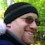## Wednesday, September 06, 2006

### Three Distinct

Can you find three distinct positive integers A, B and C such that the sum of their reciprocals equals 1?

In other words: 1/A + 1/B + 1/C = 1 where A does not equal B does not equal C (and A does not equal C).

1.How about 2, 3, and 6?

1/2 + 1/3 + 1/6 =

3/6 + 2/6 + 1/6 =

6/6 =

1

2.You got it right. The interesting part is this is the only combination that works. If they weren't distinct, you could use 1/4 + 1/4 + 1/2 or 1/3 + 1/3 + 1/3. But I believe that's it for possibilities.

3.If it has to be three of them, then their average value must be 1/3. Without 2 this is impossible unless all three are 3's.

So let's consider:
1/2 + 1/x + 1/y = 1
==> 1/x + 1/y = 1/2
==> (x + y)/xy = 1/2
==> 2x + 2y = xy
==> 2x - xy = -2y
==> x(2 - y) = -2y
==> x = -2y/(2 - y).
==> to x = 2y / (y - 2)
==> x = 2 + 4/(y - 2) [the result of long division, quite useful]

Now, for x to be an integer, y - 2 must divide 4.
So y - 2 = 1, and y = 3 (and x = 6) or
y - 2 = 2, and y = 4 (and x = 4) or
y - 2 = 4, and y = 6 (and x = 3) (repeat of previous solution.

So 3,3,3 or 2,3,6 or 2,4,4 and that's it.

4.If we opened it up to negatives, we could use -2, 1, and 2

Leave your answer or, if you want to post a question of your own, send me an e-mail. Look in the about section to find my e-mail address. If it's new, I'll post it soon.

Please don't leave spam or 'Awesome blog, come visit mine' messages. I'll delete them soon after.

Enter your Email and join hundreds of others who get their Question of the Day sent right to their mailbox

The Lamplight Manor Puzz 3-D